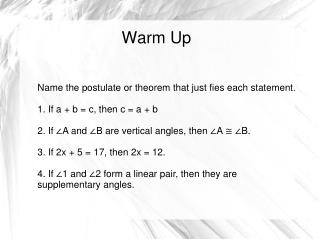# Warm Up - PowerPoint PPT PresentationDownload PresentationWarm Up

Warm Up
Download Presentation## Warm Up

- - - - - - - - - - - - - - - - - - - - - - - - - - - E N D - - - - - - - - - - - - - - - - - - - - - - - - - - -
##### Presentation Transcript

1. Warm Up Name the postulate or theorem that just fies each statement. 1. If a + b = c, then c = a + b 2. If ∠A and ∠B are vertical angles, then ∠A ≅ ∠B. 3. If 2x + 5 = 17, then 2x = 12. 4. If ∠1 and ∠2 form a linear pair, then they are supplementary angles.

2. Flow Proofs and Paragraph Proofs Students will learn how to write flow proofs and paragraphs proofs as preparatory activities to writing formal 2-column proofs.

3. The Point • Not something you're going to be tested on • An aid to writing a formal 2-column proof • If you like writing, the paragraph proof may be easier for you to start with. • If you are a visual person, the flow proof may be easier to start with. • It may turn out that just starting with the 2-column proof is easier. That's fine. You don't have to use these strategies (except for today).

4. The Problem Given: WX = YZ Prove: WY = XZ W X Y Z

5. Paragraph Proof Given: WX = YZ Prove: WY = XZ W X Y Z I know that WX = YZ because it's given. And obviously I know that XY = XY, because everything is equal to itself (that's the reflexive property of equality). So then I can take my WX = YZ and add XY to both sides—that's the addition property of equality. And I end up with WX + XY = YZ + XY. But the Segment Addition Postulate tells me that WX + XY = WY, and also that YZ + XY = XZ. Now I just put those all together, using substitution: WY = XZ. And I'm done!

6. Flow Proof Given: WX = YZ Prove: WY = XZ W X Y Z WX + XY = WY Seg Addition Post WX = YZ WX + XY = YZ + XY WY = XZ Given Addition POE Substitution YZ + XY = XZ Seg Addition Post

7. Another Example Given: ∠1 = 70° Prove: ∠2 = 110° 2 1

8. Paragraph Proof Given: ∠1 = 70° Prove: ∠2 = 110° 2 1 I know that ∠1 = 70°, because it's given. And I can see from the diagram that ∠1 and ∠2 are a linear pair (so that's given as well). If ∠1 and ∠2 are a linear pair, then they are supplementary. That's the Linear Pair Postulate. And if ∠1 and ∠2 are supplementary, then m∠1 + m∠2 = 180°. But then by the Subtraction POE, m∠2 = 180 – m∠1. So m∠2 = 180 – 70 (that's Substitution POE), and therefore m∠2 = 110°. Done!

9. Flow Proof Given: m∠1 = 70° Prove: m∠2 = 110° 2 1 ∠1 and ∠2 are an LP m∠2 = 180 - m∠1 ∠1 and ∠2 are supplementary m∠1 = 70° m∠2 = 180 - 70 m∠2 = 110 m∠1 + m∠2 = 180

10. You Try Given: ∠1 and ∠2 are complementary. ∠1 ≅ ∠3, ∠2 ≅ ∠4 Prove: ∠3 and ∠4 are complementary. 3 2 4 1

11. Paragraph Proofs Given: ∠1 and ∠2 are complementary. ∠1 ≅ ∠3, ∠2 ≅ ∠4 Prove: ∠3 and ∠4 are complementary. 3 2 4 1 We know that ∠1 and ∠2 are complementary, because it's given. That means that m∠1 + m∠2 = 90, because that's the definition of complementary angles. But it's given that ∠1 ≅ ∠3, so their angle measures are equal by the definition of congruence. Similarly, ∠2 ≅ ∠4, so their measures are equal as well. But then we can substitute m∠3 for m∠1 and m∠2 for m∠4, and we get m∠3 + m∠4 = 90. And therefore, by the definition of complementary angles, ∠3 and ∠4 are complementary.

12. Flow Proofs ∠1 ≅ ∠3 m∠1 = m∠3 ∠1 and ∠2 are complementary m∠1 + m∠2 = 90 Defn of Congruence Given m∠2 = m∠4 ∠2 ≅ ∠4 Defn of comp ∠'s Given ∠3 and ∠4 are complementary m∠3 + m∠4 = 90 Substitution Defn of comp ∠'s